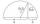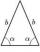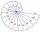Area of RT

Calculate the area of a right triangle which hypotenuse has length 10 and one hypotenuse segment has lenght 5.

Result

A =  25

Solution:Leave us a comment of example and its solution (i.e. if it is still somewhat unclear...):Be the first to comment!Next similar examples:

1. Euklid4Legs of a right triangle have dimensions 244 m and 246 m. Calculate the length of the hypotenuse and the height of this right triangle.
2. Right triangleRight triangle ABC with side a = 19 and the area S = 95. Calculate the length of the remaining sides.
3. Chord 2Point A has distance 13 cm from the center of the circle with radius r = 5 cm. Calculate the length of the chord connecting the points T1 and T2 of contact of tangents led from point A to the circle.
4. SAS triangleThe triangle has two sides long 7 and 19 and included angle 36°. Calculate area of this triangle.
5. TriangleThe triangle has known all three sides: a=5.5 m, b=5.3 m, c= 7.8 m. Calculate area of ​this triangle.
6. Right 24Right isosceles triangle has an altitude x drawn from the right angle to the hypotenuse dividing it into 2 unequal segments. The length of one segment is 5 cm. What is the area of the triangle? Thank you.
7. Area of RTIn the right triangle has orthogonal projections of legs to the hypotenuse lengths 7 cm and 12 cm. Determine the area of ​​this triangle.
8. Euclid 5Calculate the length of remain sides of a right triangle ABC if a = 7 cm and height vc = 5 cm.
9. Triangle ABCRight triangle ABC with right angle at the C, |BC|=18, |AB|=33. Calculate the height of the triangle hAB to the side AB.
10. Geometric meanCalculate the geometric mean of numbers a=15.2 and b=25.6. Determine the mean by construction where a and b are the length of the lines.
11. Oil rigOil drilling rig is 23 meters height and fix the ropes which ends are 7 meters away from the foot of the tower. How long are these ropes?
12. Euclid1Right triangle has hypotenuse c = 27 cm. How large sections cuts height hc=3 cm on the hypotenuse c?
13. Triangle TBCTBC is isosceles triangle with base TB with base angle 63° and legs length |TC| = |BC| = 25. How long is the base TB?
14. Center traverseIt is true that the middle traverse bisects the triangle?
15. CalculationHow much is sum of square root of six and the square root of 225?
16. Theorem proveWe want to prove the sentence: If the natural number n is divisible by six, then n is divisible by three. From what assumption we started?
17. RootUse law of square roots roots: ?